# Cypripedium candidum function-based MPMs

In this vignette, we will use the cypdata dataset to illustrate the estimation of function-based MPMs. To reduce file size, we have prevented some statements from running if they produce long stretches of output. Examples include most summary() calls - to run these statements, eliminate the leading hashtags and then run them. We have also written this vignette using the default Ehrlén format for all historical MPMs. All examples can be run in deVries format as well. This vignette is only a sample analysis. Detailed information and instructions on using lefko3 are available through a free online e-book called lefko3: a gentle introduction, available on the projects page of the Shefferson lab website.

### ORGANISM AND POPULATION

The white lady’s slipper, Cypripedium candidum, is a rare and long-lived North American perennial in the orchid family. The study population is located within a state nature preserve located in northeastern Illinois, USA. The population was monitored annually from 2004 to 2009, with two monitoring sessions per year. Monitoring sessions included complete censuses of the population divided into sections referred to as patches. Each monitoring session consisted of searches for previously recorded individuals, which were located according to coordinates relative to fixed stakes at the site, followed by a search for new individuals. Data recorded per individual included: location, number of non-flowering sprouts (also refered to as non-flowering stems), number of flowering sprouts, number of flowers per sprout, and number of fruits per sprout. Location was used to infer individual identity. More information about this population and its characteristics is given in Shefferson et al. (2001) and Shefferson, Mizuta, and Hutchings (2017).

### BASIC WORKFLOW

Population matrix projection modeling requires an appropriate life history model showing how all stages and transitions are related. The figure below shows a very general life history model detailing these relationships in Cypripedium candidum. The first stage of life is the dormant seed, although a seed may germinate in the year following seed production, in which case the first stage of life is the protocorm. The protocorm is the first germinated stage - an underground, mycoheterotrophic stage found only in the families Orchidaceae and Pyrolaceae. There are three years of protocorm stages, followed by a seedling stage, and finally a set of stages that comprise the size-classified adult portion of life. The figure shows 49 such stages, each for a different number of stems (including zero for vegetative dormancy) and one of two reproductive statuses.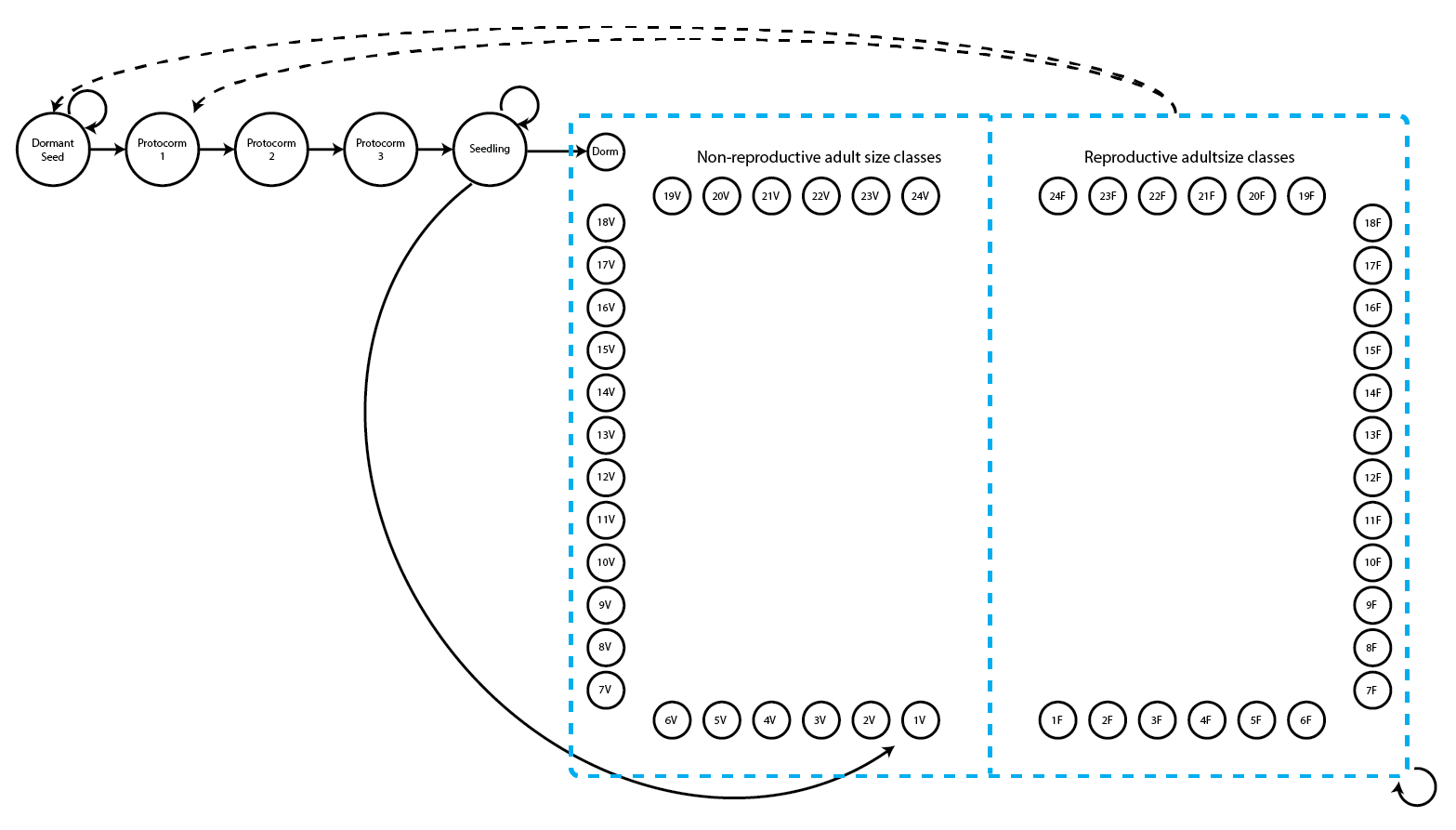Figure 5.1. Life history model of Cypripedium candidum. Survival and fecundity transitions are indicated with solid and dashed arrows, respectively.

In this figure, the juvenile stages are limited to simple, progressive transitions. New recruits may enter the population directly from a seed produced the previous year, in which case they start in the protocorm 1 stage, or they may begin as dormant seed. Dormant seed may remain dormant, die, or germinate into the protocorm 1 stage. Protocorms exist for up to three years, yielding the protocorm 1, 2, and 3 stages. Protocorm 3 leads to the seedling stage, in which the plant may persist for many years before becoming mature. The first mature stage is usually either vegetative dormancy (Dorm), during which time the plant does not sprout, or a small, non-flowering adult (1V, which has a single stem). Once in this portion of the life history, the plant may transition among 49 mature stages, including vegetative dormancy, 1-24 stems without flowers, or 1-24 stems with at least one flower.

Let’s load the dataset, which is called cypdata. The horizontal dataset cypdata and the ahistorical vertical dataset cypvert are the same data but are structured differently. Both include only data for the adult stages, and so later we will need to set juvenile transitions to constants from the literature.

data(cypdata)
#summary(cypdata)

#### Step 1. Life history model development

We will first describe the life history characterizing the population, matching it to our dataset with a stageframe for our Cypripedium candidum dataset. Since this analysis will be function-based and all sizes have reasonable representation, we will include all observed size classes here. Size is count-based, and refers to all numbers of stems that occurred, representing the life history diagram shown previously. Note that we are using the midpoint approach to determining the size bins here, using the default bin halfwidths of 0.5 (since all size bins are using this default, we do not include a binhalfwidth vector option in the sf_create() input). However, we could have used the sizemin and sizemax options to more deliberately set the size bin minima and maxima instead.

sizevector <- c(0, 0, 0, 0, 0, seq(from = 0, t = 24), seq(from = 1, to = 24))
stagevector <- c("SD", "P1", "P2", "P3", "SL", "D", "V1", "V2", "V3", "V4",
"V5", "V6", "V7", "V8", "V9", "V10", "V11", "V12", "V13", "V14", "V15", "V16",
"V17", "V18", "V19", "V20", "V21", "V22", "V23", "V24", "F1", "F2", "F3",
"F4", "F5", "F6", "F7", "F8", "F9", "F10", "F11", "F12", "F13", "F14", "F15",
"F16", "F17", "F18", "F19", "F20", "F21", "F22", "F23", "F24")
repvector <- c(0, 0, 0, 0, 0, rep(0, 25), rep(1, 24))
obsvector <- c(0, 0, 0, 0, 0, 0, rep(1, 48))
matvector <- c(0, 0, 0, 0, 0, rep(1, 49))
immvector <- c(0, 1, 1, 1, 1, rep(0, 49))
propvector <- c(1, rep(0, 53))
indataset <- c(0, 0, 0, 0, 0, rep(1, 49))
comments <- c("Dormant seed", "Yr1 protocorm", "Yr2 protocorm", "Yr3 protocorm",
cypframe <- sf_create(sizes = sizevector, stagenames = stagevector,
repstatus = repvector, obsstatus = obsvector, matstatus = matvector,
propstatus = propvector, immstatus = immvector, indataset = indataset,
#cypframe

The resulting object, cypframe, shows a data frame that includes the following information in order for each stage: the stage’s name; primary, secondary, and tertiary size; minimum and maximum ages associated with the stage; reproductive status; status as observable; status as a propagule; status as immature; status as mature; whether it occurs in the dataset; half-width, minimum, maximum, centroid, and full width of primary, secondary, and tertiary size class bins; stage group number; and a comments field. We used one size variable, so values for the secondary and tertiary sizes are set to NA. Stage names and combinations of characteristics occurring within the dataset must be unique to prevent estimation errors, and the comments field may be edited to include any information deemed pertinent.

#### Step 2a. Data standardization

Now we will standardize our vertical dataset into a historically-formatted vertical (hfv) data frame using the verticalize3() function. The resulting dataset will have each individual’s observed life history broken up into states corresponding to three consecutive monitoring occasions (years) per row, with plant identity marked in each row.

vertdata <- verticalize3(data = cypdata, noyears = 6, firstyear = 2004,
patchidcol = "patch", individcol = "plantid", blocksize = 4,
sizeacol = "Inf2.04", sizebcol = "Inf.04", sizeccol = "Veg.04",
repstracol = "Inf.04", repstrbcol = "Inf2.04", fecacol = "Pod.04",
stageassign = cypframe, stagesize = "sizeadded", NAas0 = TRUE)
summary_hfv(vertdata, full = FALSE)
#>
#> This hfv dataset contains 320 rows, 57 variables, 1 population,
#> 3 patches, 74 individuals, and 5 time steps.

In the above code, we described the input dataset in a way that allows R to reorganize it appropriately. In the input to the function, we utilized a repeating pattern of variable names arranged in the same order for each monitoring occasion. This arrangement allows us to enter only the first variable in each set, as long as noyears and blocksize are set properly and no gaps or shuffles appear among columns in the dataset. lefko3’s data management functions do not require such repeating patterns, but they do make the required function input much more succinct. Without these patterns, column names should be input as vectors in the same temporal order for each input option, and the blocksize argument should be omitted.

The output dataset includes a number of summary variables (to see the variables please remove full = FALSE from the summary_hfv() call), but the data is broken down into groups of three consecutive monitoring occasions per row (occasions t+1, t, and t-1, corresponding to year3, year2, and year1 in the output, respectively), with individuals spread across multiple rows. The output dataset is further limited to those entries in which the individual is alive in occasion t (year2), meaning that all rows in which an individual is dead or not yet recruited in occasion t were dropped. We end up with 320 rows of data and 57 variables.

This reorganized dataset includes the sizeadded group of three variables, which were automatically calculated. These are sums of the size variables for each occasion, such that size1added is calculated as sizea1 + sizeb1 + sizec1. This term may or may not make sense depending on the dataset. Here, the full size of the individual in each occasion equals this sum, because size is determined as the number of stems per plant, and the three original size columns give the numbers of three different kinds of stems per plant. Since size is given as the total number of stems in this example, we will use the sizeadded group of variables to code individual size.

Size and fecundity are both count variables here, and we need to determine the appropriate distribution to use in both cases. Let’s look at histograms of size and fecundity in occasion t.

par(mfrow=c(1,2))
hist(vertdata$size2added, main = "Size in time t", xlab = "No. of stems in time t") hist(subset(vertdata, repstatus2 == 1)$feca2, main = "Fecundity in time t",
xlab = "No. of fruit pods in time t")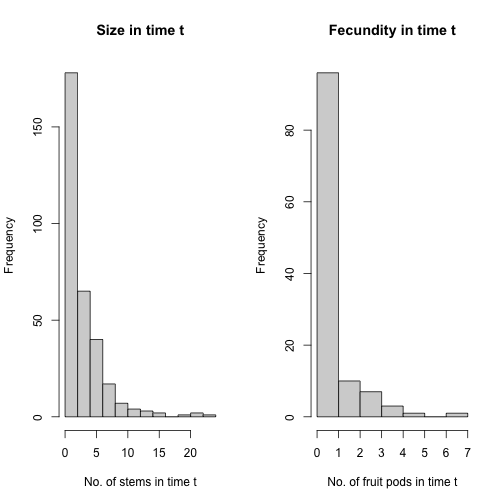Figure 5.2. Histogram of size and fecundity in occasion t

Stem number has a very low mean, and the curve declines fairly smoothly. The Poisson or negative binomial distribution might work for size. Fecundity appears to have many zeros, suggesting that we might need a zero-inflated count distribution. Let’s conduct a formal set of tests to determine the appropriate distributions for size and fecundity, using a function that checks for the appropriate distributions for all possible vital rate functions in our dataset.

hfv_qc(data = vertdata, vitalrates = c("surv", "obs", "size", "repst", "fec"),
#> Survival:
#>
#>   Data subset has 58 variables and 320 transitions.
#>
#>   Variable alive3 has 0 missing values.
#>   Variable alive3 is a binomial variable.
#>
#>
#> Observation status:
#>
#>   Data subset has 58 variables and 303 transitions.
#>
#>   Variable obsstatus3 has 0 missing values.
#>   Variable obsstatus3 is a binomial variable.
#>
#>
#> Primary size:
#>
#>   Data subset has 58 variables and 288 transitions.
#>
#>   Variable size3added has 0 missing values.
#>   Variable size3added appears to be an integer variable.
#>
#>   Variable size3added is fully positive, lacking even 0s.
#>
#>   Overdispersion test:
#>     The variance in size3added is 13.41
#>     The probability of this dispersion level by chance assuming that
#>     and an alternative hypothesis of overdispersion, is 3.721e-138
#>     Variable size3added is significantly overdispersed.
#>
#>   Zero-inflation and truncation tests:
#>     Mean lambda in size3added is 0.02592
#>     The actual number of 0s in size3added is 0
#>     The expected number of 0s in size3added under the null hypothesis is 7.465
#>     The probability of this deviation in 0s from expectation by chance is 0.9964
#>     Variable size3added is not significantly zero-inflated.
#>
#>     Variable size3added does not include 0s, suggesting that a zero-truncated distribution may be warranted.
#>
#>
#> Reproductive status:
#>
#>   Data subset has 58 variables and 288 transitions.
#>
#>   Variable repstatus3 has 0 missing values.
#>   Variable repstatus3 is a binomial variable.
#>
#>
#> Fecundity:
#>
#>   Data subset has 58 variables and 118 transitions.
#>
#>   Variable feca2 has 0 missing values.
#>   Variable feca2 appears to be an integer variable.
#>
#>   Variable feca2 is fully non-negative.
#>
#>   Overdispersion test:
#>     Mean feca2 is 0.7881
#>     The variance in feca2 is 1.536
#>     The probability of this dispersion level by chance assuming that
#>     the true mean feca2 = variance in feca2,
#>     and an alternative hypothesis of overdispersion, is 0.1193
#>     Dispersion level in feca2 matches expectation.
#>
#>   Zero-inflation and truncation tests:
#>     Mean lambda in feca2 is 0.4547
#>     The actual number of 0s in feca2 is 68
#>     The expected number of 0s in feca2 under the null hypothesis is 53.65
#>     The probability of this deviation in 0s from expectation by chance is 5.904e-06
#>     Variable feca2 is significantly zero-inflated.

The results of these tests show us that size is overdispersed and zero-truncated (the zero truncation is due to the fact that we observed instances of size equal to zero within the vegetative dormancy stage). We will use the zero-truncated negative binomial distribution for this vital rate. Our tests of fecundity show no significant overdispersion, but the number of zeros exceeds expectation, suggesting that we use a zero-inflated Poisson distribution. All other vital rates appear to match expectation for the binomial distribution.

#### Step 2b. Vertical dataset standardization

We may also wish to see how to proceed if our original dataset is already in vertical, ahistorical format, or a format in which each row gives the state of an individual in a single monitoring occasion. This package also includes dataset cypvert, which is the same dataset as cypdata but set in ahistorical vertical format. Here, we use the historicalize3() function to deal with this dataset.

data(cypvert)
dim(cypdata)
#>  77 29
dim(cypvert)
#>  322  14
#summary(cypvert)

This dataset has more rows and fewer columns, because we constructed the dataset by splitting the data for each individual across multiple rows. After three columns of identifying information (plantid, patch, and censor), a single column designates the time of occasion t, given as year2. This dataset then includes columns showing individual state in pairs of consecutive years corresponding to occasions t and t+1. State in occasion t-1 is not presented because this is an ahistorical dataset. This dataset includes the plantid variable, which is an individual identity term that allows us to associate rows with their individuals and so allows conversion. The historicalize3() function uses individual identity to reorganize datasets into historical vertical format.

vertdata2 <- historicalize3(data = cypvert, patchidcol = "patch",
individcol = "plantid", year2col = "year2", sizea2col = "Inf2.2",
sizea3col = "Inf2.3", sizeb2col = "Inf.2", sizeb3col = "Inf.3",
sizec2col = "Veg.2", sizec3col = "Veg.3", repstra2col = "Inf2.2",
repstra3col = "Inf2.3", repstrb2col = "Inf.2", repstrb3col = "Inf.3",
feca2col = "Pod.2", feca3col = "Pod.3", repstrrel = 2, stageassign = cypframe,
stagesize = "sizeadded", censorcol = "censor", censorkeep = 1, censor = FALSE,
NAas0 = TRUE, reduce = TRUE)
summary_hfv(vertdata2, full = FALSE)
#>
#> This hfv dataset contains 320 rows, 57 variables, 1 population,
#> 3 patches, 74 individuals, and 5 time steps.

Comparing the dimensions of the new vertical datasets, we find that the lengths of the datasets are the same in terms of rows and columns, and the variables and data are the same although the order of the columns and rows might not match (see the summaries for comparison).

One important consideration is the use of censor variables. Censoring a demographic dataset refers to the elimination of suspect data points from analysis. This is typically accomplished by including a binary variable in the dataset denoting whether an individual datum is to be kept or excluded. The objects vertdata and vertdata2 were both created without censoring. However, because the datasets included censor variables (all data were set to be included, with no suspect data), we wished to incorporate those variables in the final datasets. Hence, although censor = FALSE in both the call to verticalize3() and the call to historicalize3(), we also noted censorcol = "censor" and censorkeep = 1 in the call to historicalize3() (the latter option specifies the value of the censor variable coding for a “good” datum). Failing to add these options to the call to historicalize3() will produce approximately the same dataset, but with some zeroes entering variables censor1, censor2, and censor3 in the historicalized dataset that do not exist in the verticalized dataset.

#### Step 2c. Provide supplemental information for matrix estimation

The next steps involve adding some external data to parameterize the matrices properly. The supplemental() function provides a means of inputting four kinds of data into MPM construction:

1. fixed transition values derived from other studies and added as constants to matrices;
2. proxy transition values when data for particular transitions do not exist and other, estimable transitions will be used as proxies;
3. reproductive multipliers to indicate which stages lead to the production of which stages, and at what level relative to estimated fecundity; and
4. survival multipliers, in cases in which proxy survival transitions are used but must be set at elevated or reduced levels relative to the original transition.

We will create two supplement tables, the first covering the historical analysis, and the second covering the ahistorical analysis. Each row refers to a specific transition, and in the historical case there are codes for 23 given transitions (12 for the ahistorical case). The first nine of the historical transitions are set to specific probabilities (six for the ahistorical), and the next 12 are transitions that will be set to other, estimated transitions acting as proxies (four for the ahistorical; these are the non-NA transitions in eststage set below). The final two terms are fecundity multipliers. Based on the literature, the proxies for entry into the adult classes are transitions from dormancy, as below. Where necessary, we also use rep and mat as shorthand to code for all reproductive stages and all mature stages, respectively.

cypsupp3 <- supplemental(stage3 = c("SD", "SD", "P1", "P1", "P2", "P3", "SL",
"SL", "SL", "D", "V1", "V2", "V3", "D", "V1", "V2", "V3", "mat", "mat",
"mat", "mat", "SD", "P1"),
stage2 = c("SD", "SD", "SD", "SD", "P1", "P2", "P3", "SL", "SL", "SL", "SL",
"SL", "SL", "SL", "SL", "SL", "SL", "D", "V1", "V2", "V3", "rep", "rep"),
stage1 = c("SD", "rep", "SD", "rep", "SD", "P1", "P2", "P3", "SL", "P3", "P3",
"P3", "P3", "SL", "SL", "SL", "SL", "SL", "SL", "SL", "SL", "mat", "mat"),
eststage3 = c(NA, NA, NA, NA, NA, NA, NA, NA, NA, "D", "V1", "V2", "V3", "D",
"V1", "V2", "V3", "mat", "mat", "mat", "mat", NA, NA),
eststage2 = c(NA, NA, NA, NA, NA, NA, NA, NA, NA, "D", "D", "D", "D", "D",
"D", "D", "D", "D", "V1", "V2", "V3", NA, NA),
eststage1 = c(NA, NA, NA, NA, NA, NA, NA, NA, NA, "D", "D", "D", "D", "D",
"D", "D", "D", "V1", "V1", "V1", "V1", NA, NA),
givenrate = c(0.08, 0.08, 0.1, 0.1, 0.1, 0.1, 0.125, 0.2, 0.2, NA, NA, NA, NA,
NA, NA, NA, NA, NA, NA, NA, NA, NA, NA),
multiplier = c(NA, NA, NA, NA, NA, NA, NA, NA, NA, NA, NA, NA, NA, NA, NA, NA,
NA, 1, 1, 1, 1, 0.5, 0.5),
type = c("S", "S", "S", "S", "S", "S", "S", "S", "S", "S", "S", "S", "S", "S",
"S", "S", "S", "S", "S", "S", "S", "R", "R"),
type_t12 = c("S", "F", "S", "F", "S", "S", "S", "S", "S", "S", "S", "S", "S",
"S", "S", "S", "S", "S", "S", "S", "S", "S", "S"), stageframe = cypframe)

cypsupp2 <- supplemental(stage3 = c("SD", "P1", "P2", "P3", "SL", "SL", "D",
"V1", "V2", "V3", "SD", "P1"),
stage2 = c("SD", "SD", "P1", "P2", "P3", "SL", "SL", "SL", "SL", "SL", "rep",
"rep"),
eststage3 = c(NA, NA, NA, NA, NA, NA, "D", "V1", "V2", "V3", NA, NA),
eststage2 = c(NA, NA, NA, NA, NA, NA, "D", "D", "D", "D", NA, NA),
givenrate = c(0.08, 0.1, 0.1, 0.1, 0.125, 0.2, NA, NA, NA, NA, NA, NA),
multiplier = c(NA, NA, NA, NA, NA, NA, NA, NA, NA, NA, 0.5, 0.5),
type = c("S", "S", "S", "S", "S", "S", "S", "S", "S", "S", "R", "R"),
stageframe = cypframe, historical = FALSE)
#cypsupp3
#cypsupp2

#### Step 3. Tests of history, and vital rate modeling

Matrix estimation can proceed either via raw matrix methods, as initially outlined in Ehrlén (2000), or via function-based matrix development, essentially equivalent to the creation of discretized complex integral projection models per Ellner and Rees (2006) and as further described in the non-Gaussian case in Shefferson, Warren II, and Pulliam (2014). In the raw MPM case, no vital rate models are estimated, whereas in function-based MPMs, vital rates are first analyzed via linear or non-linear models of the raw demographic dataset, and then functions are created that estimate these vital rates according the inputs given. Matrices are then estimated using these functions, as opposed to the raw data.

#### Step 3a. General modeling strategy

Most function-based matrices, whether integral projection models or otherwise, are developed using either a generalized linear modeling (GLM) strategy, or a generalized linear mixed modeling (GLMM, or mixed) strategy. The former is more common and simpler, but the latter is more statistically sound because it corrects the lack of independence in data originating from repeated sampling of the same individuals. The difference between the two methods with regards to vital rate modeling is strongly related to assumptions regarding individual and spatiotemporal variation in vital rates.

In both GLM and GLMM-based MPMs, the underlying dataset utilized is a vertical dataset. Each row of data gives the state of the individual in either two consecutive occasions (the ahistorical case), or three consecutive occasions (the historical case). Under the GLM framework, time in occasion t is a fixed categorical variable, and individual identity is ignored. Treating time as fixed implies that the actual monitoring occasions are the only times for which inference is sought. Thus, it would be inappropriate to infer future population dynamics after 2009 for a dataset collected between 2004 and 2009. Ignoring individual identity treats all transitions as independent, even though data originating from the same sampled individual is not independent of that individual’s previous or future transitions. This statistical dependence may inflate Type 1 error in the linear modeling, yielding more significant terms in the chosen best-fit model and causing the retention of more terms than is warranted.

Under the GLMM (generalized linear mixed model, or mixed) framework, both time and individual identity are typically treated as random categorical terms. This has two major implications. First, both time and individuals can be assumed to be random samples from a broader population of times and individuals for which inference is sought. Thus, sampled monitoring occasions represent a greater universe of years for which inference can be made. Second, treating individual as a random categorical term eliminates the pseudoreplication that is inherent in the GLM approach when individuals are monitored potentially many times, because each individual is assumed to be randomly drawn and associated with its own response distribution. Subpopulations may also be considered random, in which case they are assumed to have been sampled from all possible subpopulations whether or not other subpopulations actually exist. We will use this approach in this vignette.

#### Step 3b. Size and fecundity distributions

The next step is to choose the underlying distributions. The probabilities of survival, observation, reproductive status, and maturity status are set to the binomial distribution, and this cannot be altered. Size transition probability and fecundity rate can be set to the Gaussian, Gamma, Poisson, or negative binomial distributions, with zero-inflated and zero-truncated versions of the Poisson and negative binomial also available. If size or fecundity rate is continuous, then it should be set to the Gaussian distribution if not too right-skewed; otherwise, the Gamma distribution may be better (although our implementation of the Gamma distribution currently requires fully positive data). If size or fecundity is a count variable, then it should be set to the Poisson distribution if the mean equals the variance. The negative binomial distribution is provided in cases where the assumption that the mean equals the variance is clearly broken.

#### Step 3c. Model building and selection

In lefko3, function modelsearch() is the workhorse that conducts vital rate model estimation. Here, we will create a full suite of vital rate models for the Cypripedium candidum dataset. Before proceeding, we still need to decide on the the correct vital rates to model, the proper statistical distributions for estimated vital rates, the proper parameterization of each vital rate, and the strategy for determination of the best-fit model for each vital rate.

Function modelsearch() estimates up to fourteen vital rate models:

1. survival probability from occasion t to occasion t+1,

2. observation probability in occasion t+1 assuming survival until that time,

3. primary size in occasion t+1 assuming survival and observation in that time,

4. secondary size in occasion t+1 assuming survival and observation in that time,

5. tertiary size in occasion t+1 assuming survival and observation in that time,

6. reproduction status in occasion t+1 assuming survival and observation until that time,

7. fecundity rate assuming survival until and observation and reproduction in the occasion of production of offspring (occasion t or t+1; mature only),

8. juvenile survival probability from occasion t to occasion t+1,

9. juvenile observation probability in occasion t+1 assuming survival until that time,

10. juvenile primary size in occasion t+1 assuming survival and observation in that time,

11. juvenile secondary size in occasion t+1 assuming survival and observation in that time,

12. juvenile tertiary size in occasion t+1 assuming survival and observation in that time,

13. reproduction status in occasion t+1 assuming survival and observation until that time of a juvenile in occasion t that is becoming mature in occasion t+1, and

14. maturity status in occasion t+1 assuming survival until that time of a juvenile in occasion t.

The default settings for modelsearch estimate 1) survival probability, 3) primary size distribution, and 7) fecundity, which are the minimum three vital rates required for a full MPM. Observation probability (option obs in vitalrates) should only be included when a life history stage or size exists that cannot be observed. For example, in the case of a plant with vegetative dormancy, the observation probability can be thought of as the sprouting probability, which is a biologically meaningful vital rate (Shefferson et al. 2001). Reproduction status (option repst in vitalrates) should only be modeled if size classification needs to be stratified by the ability to reproduce, as when equivalently sized stages exist differing in the ability to reproduce. Secondary and tertiary size should only be modeled when two or three size variables are used in stage classification. Since Cypripedium candidum is capable of long bouts of vegetative dormancy, since we wish to stratify the population into reproductive and non-reproductive adults of the same size classes, and since we have no data derived from juvenile individuals, we will set vitalrates = c("surv", "obs", "size", "repst", "fec") and leave the juvenile-related options at their defaults.

Next, we need to set the proper statistical distribution for size and fecundity. Size was measured as the number of stems and so is a count variable. Likewise, fecundity is actually estimated as the number of fruits produced per plant, and so is also a count variable. We have already performed tests for overdispersion and zero-inflation, and we are also aware that size in observed stages cannot equal 0, requiring zero truncation in that parameter. So we will set size to the zero-truncated negative binomial distribution, and fecundity to the zero-inflated Poisson distribution.

Now we need the proper model parameterizations for each vital rate, using the suite option. The default, suite = "main", under the mixed model setting (approach = "mixed") starts with the estimation of global models that include size and reproductive status in occasions t and t-1 as fixed factors, with individual identity and time in occasion t (year t) set as random categorical terms. Other terms can be specified, including individual covariates, spatial density, and age. Setting suite = "full" will yield global models that also include all two-way interactions. The default under the GLM setting (approach = "glm") makes time in occasion t a fixed term and drops individual identity from consideration. The global model under suite = "full" then includes all fixed factors noted before, plus all two-way interactions between fixed factors ("full" is the only setting with interaction terms). If the population is not stratified by reproductive status, then suite = "size" will eliminate reproductive status terms and use all others in the global model. If size is not important, then suite = "rep" will eliminate size but keep reproductive status and all other terms. Finally, suite = "cons" will result in a global model in which neither reproductive status nor size are considered, although optional terms such as age or spatial density may be still be incorporated. We will set suite = "full".

Finally, we need to determine the proper strategy for the determination of the best-fit model. Model building proceeds through the dredge function in package MuMIn (Bartoń 2014), and each model has an associated AICc value. The default setting in lefko3 (bestfit = "AICc&k") will compare all models within 2.0 AICc units of the model with $$\Delta AICc = 0$$, and choose the one with the lowest degrees of freedom. This approach is generally better than the alternative, which simply uses the model with $$\Delta AICc = 0$$ (bestfit = "AICc"), as all models within 2.0 AICc units of that model are equally parsimonious and so fewer degrees of freedom result from fewer parameters estimated (Burnham and Anderson 2002).

cypmodels3 <- modelsearch(vertdata, historical = TRUE, approach = "mixed",
vitalrates = c("surv", "obs", "size", "repst", "fec"), sizedist = "negbin",
size.trunc = TRUE, fecdist = "poisson", fec.zero = TRUE, suite = "full",
#>
#> Developing global model of survival probability...
#>
#> Global model of survival probability developed. Proceeding with model dredge...
#>
#> Developing global model of observation probability...
#>
#> Global model of observation probability developed. Proceeding with model dredge...
#>
#> Developing global model of primary size...
#>
#> Global model of primary size developed. Proceeding with model dredge...
#>
#> Developing global model of reproduction probability...
#>
#> Global model of reproduction probability developed. Proceeding with model dredge...
#>
#> Developing global model of fecundity...
#>
#> Global model of fecundity developed. Proceeding with model dredge...
#>
#> Finished selecting best-fit models.
#summary(cypmodels3)

As modelsearch works, it produces text describing its current processes and any issues encountered. It is quite likely that many warning messages will appear, and these may be of use in understanding the dataset and how well it conforms to analytical assumptions. We have silenced most of this with the quiet = "partial" option, although some red text still indicates mileposts reached by the function and a few warnings that have slipped through from the core estimation functions. The warnings are not to be terribly concerned about. However, we encourage users to allow the function to run unsilenced in case of severe modeling problems (quiet = FALSE). Please read the documentation for functions lm() (stats package), glm() (stats package), glm.nb() (MASS package), lmer() (lme4 package), glmer() (lme4 package), glmmTMB() (glmmTMB package), zeroinfl() (pscl package), vglm() (VGAM package), and dredge() (MuMIn package) for further information on the sources of warnings.

In the output, we see historical terms determining primary size, suggesting that we cannot ignore history. As such, the models created above are actually only usable for the construction of historical models. We can also see that although the accuracy of our some vital rate models is very high, while it was only fair for others. For comparison, we may wish to estimate ahistorical models. In that case, we also need linear models in which the global models tested do not include state at occasion t-1, as below.

cypmodels2 <- modelsearch(vertdata, historical = FALSE, approach = "mixed",
vitalrates = c("surv", "obs", "size", "repst", "fec"), sizedist = "negbin",
size.trunc = TRUE, fecdist = "poisson", fec.zero = TRUE, suite = "full",
#>
#> Developing global model of survival probability...
#>
#> Global model of survival probability developed. Proceeding with model dredge...
#>
#> Developing global model of observation probability...
#>
#> Global model of observation probability developed. Proceeding with model dredge...
#>
#> Developing global model of primary size...
#>
#> Global model of primary size developed. Proceeding with model dredge...
#>
#> Developing global model of reproduction probability...
#>
#> Global model of reproduction probability developed. Proceeding with model dredge...
#>
#> Developing global model of fecundity...
#>
#> Global model of fecundity developed. Proceeding with model dredge...
#>
#> Finished selecting best-fit models.
#summary(cypmodels2)

Fewer models were estimated per dredge, since fewer parameters were tested in the global models (size and reproductive status in occasion t-1 were not included). Since historical terms were not tested, the best-fit model for primary size looks quite different. A thorough comparison shows that many of the best-fit models are similar between historical and ahistorical analysis, and that the only model different appears to be the primary size model.

#### Step 4. MPM estimation

Now we will create function-based MPMs. Let’s first create a set of ahistorical matrices.

cypmatrix2 <- flefko2(stageframe = cypframe, supplement = cypsupp2,
modelsuite = cypmodels2, data = vertdata, patch = NULL)
#summary(cypmatrix2)

A glance at the summary highlights that these are large, dense matrices - here, 2,459 out of 2,916 total elements have been estimated (84.3%). In raw matrices, elements associated with transitions from specific stages are only estimated when individuals actually move through those transitions, and so the numbers of transitions estimated and of stages identified are strongly limited by the size of the dataset. In function-based matrices, in contrast, the linear models estimated allow the estimation of all elements that are theoretically possible (i.e. only structural zeros are not estimated), and so stages may be developed for the full range of sizes experienced by individuals.

The output of the flefko2() call includes several key elements. One of the most important is a data frame showing the order of matrices produced. Let’s take a look at this object.

#cypmatrix2$labels Here we see that our five matrices are annual matrices corresponding to the same population/patch (we did not input vital rate models using patch identity as a term, so our matrices are for the whole population rather than for individual patches). Other elements in this object include summaries of the stages used, in the order that they occur in the matrix. Since this is an ahistorical MPM, the key element here is cypmatrix2$ahstages. If this were a historical MPM, then cypmatrix2$ahstages would give the order of stages, and cypmatrix2$hstages would include the order of stage-pairs corresponding to the rows and columns in such MPMs.

Next, we will create a set of historical Lefkovitch matrices. To make the resulting lefkoMat object reasonably small, we will use sparse matrices (sparse_output = TRUE).

cypmatrix3 <- flefko3(stageframe = cypframe, supplement = cypsupp3,
modelsuite = cypmodels3, data = vertdata, sparse_output = TRUE)
#summary(cypmatrix3)

We see many more total elements per matrix (over 8.5 million, in contrast to 2,916 in the ahistorical case), and many more rows and columns (54 rows and columns in the ahistorical case, and 542 = 2,916 rows and columns in the historical case). However, historical matrices are composed primarily of structural zeros. Thus, with only 117,908 + 2,352 = 120,260 elements estimated per matrix, only 1.4% of elements are non-zero. Note also that we used sparse matrix format to hold these matrices. The current size of object cypmatrix3 should be around 14 MB, whereas the equivalent object using standard matrices would be over 1 GB in size (to compare, repeat the code above without the sparse_output argument, or set it to FALSE).

Now let’s estimate the historical and ahistorical element-wise mean matrices.

tmeans2r <- lmean(cypmatrix2)
tmeans3r <- lmean(cypmatrix3)
#summary(tmeans2r)
#summary(tmeans3r)

It might help to do a more holistic comparison of the ahistorical vs. historical MPMs. Let’s create matrix plots of the ahistorical and historical means using the image3() function. Let’s first view the ahistorical matrix image.

image3(tmeans2r, used = 1)
#> []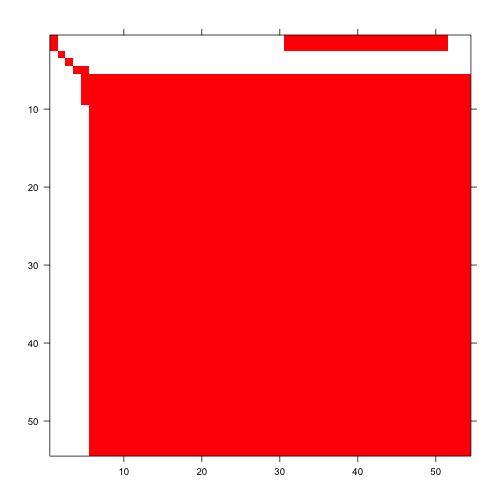Figure 5.3. Image of ahistorical element-wise matrix mean

This is a fairly large matrix, and rather dense though with obviously large clusters of zero elements particularly at the edges. Now let’s see the historical matrix.

image3(tmeans3r, used = 1)
#> []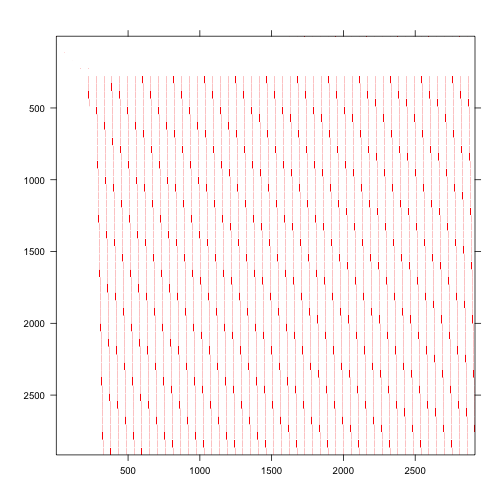Figure 5.4. Image of historical element-wise matrix mean

Historical matrices are often huge and always sparse, just as our historical matrix is in the plot above. Although there are roughly 8.5 million elements, very few are non-zero and those are identified as the streaks in the matrix image above.

For further comparison, let’s decompose the mean historical matrix into conditional historical matrices. We’ll take a look at the matrix conditional on vegetative dormancy in occasion t-1.

condm3r <- cond_hmpm(tmeans3r)
#print(condm3r$Mcond[]$D, digits = 3)

This conditional matrix takes the theoretically possible elements in which stage in time t-1 is D (vegetative dormancy), and creates a matrix with the dimensions and row and column order of an ahistorical matrix with them. Since vegetative dormancy is an adult stage, we do not see survival transitions from juvenile stages - only adult portions of the matrix are shown, including both survival and fecundity transitions.

#### Step 5. MPM analysis

Let’s now conduct some projection analyses. First, we will estimate the deterministic and stochastic population growth rates for these means. The set.seed() function makes our stochastic results reproducible.

set.seed(42)
cypann2s <- slambda3(cypmatrix2)
set.seed(42)
cypann3s <- slambda3(cypmatrix3)

lambda3(tmeans2r)
#>   pop patch    lambda
#> 1   1     1 0.9603461
lambda3(tmeans3r)
#>   pop patch    lambda
#> 1   1  <NA> 0.9605928
cypann2s
#>   pop patch           a         var         sd           se
#> 1   1     1 -0.04043205 0.002352739 0.04850504 0.0004850504
cypann3s
#>   pop patch          a         var         sd           se
#> 1   1  <NA> -0.0401626 0.002337849 0.04835131 0.0004835131

These $$\lambda$$ values are similar to one another, as are estimates of $$a = \text{log} \lambda _{S}$$. Let’s take a look at a plot of annual deterministic growth rates (stochastic growth rate is calculated as a single value across time, and so is not included). We find that annual $$\lambda$$ estimates differ only slightly between ahistorical and historical analyses.

cypann3 <- lambda3(cypmatrix3)
cypann2 <- lambda3(cypmatrix2)

plot(lambda ~ year2, data = cypann2, xlab = "Year", ylab = "Lambda",
ylim = c(0.93, 0.98), type = "l", lty= 1, lwd = 2, bty = "n")
lines(lambda ~ year2, data = cypann3, lty = 2, lwd= 2, col = "red")
legend("bottomright", c("ahistorical", "historical"), lty = c(1, 2),
col = c("black", "red"), lwd = 2, bty = "n")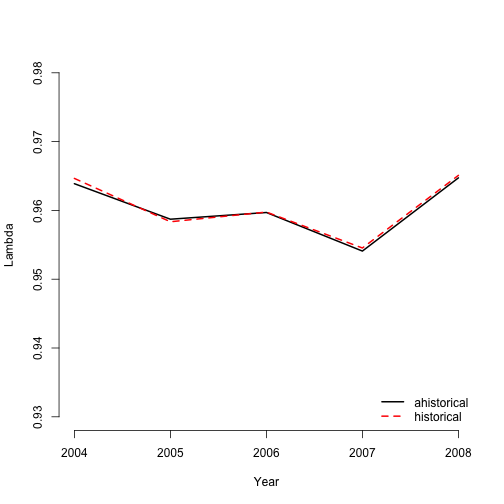Figure 5.5. Ahistorical vs. historical annual population growth rate

Now let’s take a look at the stable stage distributions. We will look at deterministic and stochastic versions of the ahistorical and historical MPMs.

tm2ss <- stablestage3(tmeans2r)
tm3ss <- stablestage3(tmeans3r)
tm2ss_s <- stablestage3(cypmatrix2, stochastic = TRUE, seed = 42)
tm3ss_s <- stablestage3(cypmatrix3, stochastic = TRUE, seed = 42)

ss_put_together <- cbind.data.frame(tm2ss$ss_prop, tm3ss$ahist$ss_prop, tm2ss_s$ss_prop, tm3ss_s$ahist$ss_prop)
names(ss_put_together) <- c("det ahist", "det hist", "sto ahist", "sto hist")
rownames(ss_put_together) <- tm2ss$stage_id barplot(t(ss_put_together), beside=T, ylab = "Proportion", xlab = "Stage", ylim = c(0, 0.15), col = c("black", "orangered", "grey", "darkred"), bty = "n") legend("topright", c("det ahist", "det hist", "sto ahist", "sto hist"), col = c("black", "orangered", "grey", "darkred"), pch = 15, bty = "n")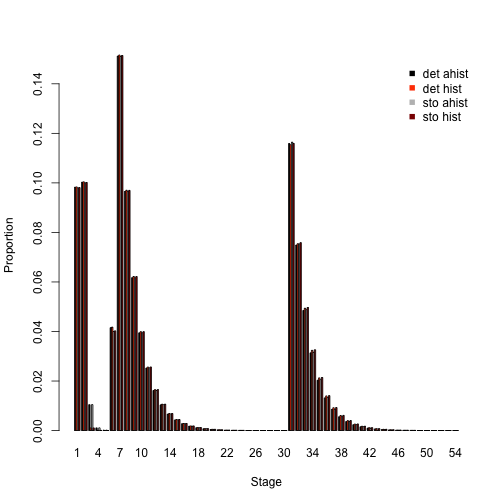Figure 5.6. Ahistorical vs. historically-corrected stable and long-run mean stage distribution Overall, these are very similar patterns. Whether ahistorical or historical, deterministic or stochastic, our analyses suggest that small non-reproductive adults take up the greatest share of the stable stage structure, with small reproductive adults coming next, and then dormant seed and 1st-year protocorms. Next let’s look at the reproductive values associated with both ahistorical and historical approaches. We will standardize each reproductive value vector to sum to 1.0 to make them comparable in a single plot (normally we would not standardize across analyses like this, using the first or minimum reproductive value in each case for standardization instead). tm2rv <- repvalue3(tmeans2r) tm3rv <- repvalue3(tmeans3r) tm2rv_s <- repvalue3(cypmatrix2, stochastic = TRUE, seed = 42) tm3rv_s <- repvalue3(cypmatrix3, stochastic = TRUE, seed = 42) rv_put_together <- cbind.data.frame((tm2rv$rep_value / sum(tm2rv$rep_value)), (tm3rv$ahist$rep_value / sum(tm3rv$ahist$rep_value)), (tm2rv_s$rep_value / sum(tm2rv_s$rep_value)), (tm3rv_s$ahist$rep_value / sum(tm3rv_s$ahist$rep_value))) names(rv_put_together) <- c("det ahist", "det hist", "sto ahist", "sto hist") rownames(rv_put_together) <- tm2rv$stage_id

barplot(t(rv_put_together), beside=T, ylab = "Relative rep value", xlab = "Stage",
ylim = c(0, 0.03), col = c("black", "orangered", "grey", "darkred"), bty = "n")
legend("topleft", c("det ahist", "det hist", "sto ahist", "sto hist"),
col = c("black", "orangered", "grey", "darkred"), pch = 15, bty = "n")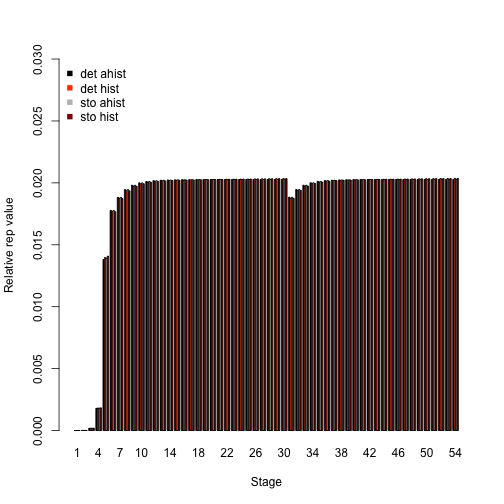Figure 5.7. Ahistorical vs. historically-corrected deterministic and stochastic reproductive values

We see roughly equivalent patterns here. All analyses show the smallest reproductive value in the juvenile stages, while adults have the greatest value, particularly medium- and large-sized adults of both flowering and non-flowering kinds. The key difference appears to be that historical analyses predict decreasing reproductive value for adults above a certain size.

Let’s now conduct a sensitivity analysis, again using both deterministic and stochastic approaches. First we will look at the ahistorical MPM.

tm2sens <- sensitivity3(tmeans2r)
set.seed(42)
tm2sens_s <- sensitivity3(cypmatrix2, stochastic = TRUE)

# The highest deterministic sensitivity value:
max(tm2sens$ah_sensmats[][which(tmeans2r$A[] > 0)])
#>  0.2002353
# This value is associated with element:
which(tm2sens$ah_sensmats[] == max(tm2sens$ah_sensmats[][which(tmeans2r$A[] > 0)])) #>  378 # The highest stochastic sensitivity value: max(tm2sens_s$ah_sensmats[][which(tmeans2r$A[] > 0)]) #>  0.1771649 # This value is associated with element: which(tm2sens_s$ah_sensmats[] ==
max(tm2sens_s$ah_sensmats[][which(tmeans2r$A[] > 0)]))
#>  1674

The highest sensitivity value in the deterministic case appears to be associated with the transition from 1-sprouted non-flowering adult to the largest flowering adult (element 378, in the 7th column and 54th row), and the highest value in the stochastic case is the transition from 1-sprouted flowering adult to the largest flowering adult (element 1674, in the 31st column and 54th row). Inspecting each sensitivity matrix also shows that transitions near that element in both matrices are also associated with rather high sensitivities.

Now let’s compare with the historical case. We will compare both the full historical sensitivity matrices, and also look at the historically-corrected deterministic sensitivities. Note that we need to convert to standard matrix format to get the correct element numbers, since the input matrices are in sparse format. We will also use a small number of time steps for the historical sensitivity analysis to make the time required more manageable.

tm3sens <- sensitivity3(tmeans3r)
set.seed(42)
tm3sens_s <- sensitivity3(cypmatrix3, stochastic = TRUE, steps = 500)

# The highest deterministic sensitivity value:
max(as.matrix(tm3sens$h_sensmats[])[which(as.matrix(tmeans3r$A[]) > 0)])
#>  0.05042798
# This value is associated with element:
which(as.matrix(tm3sens$h_sensmats[]) == max(as.matrix(tm3sens$h_sensmats[])[which(as.matrix(tmeans3r$A[]) > 0)])) #>  962658 962712 962982 963306 963522 963630 963792 # The highest stochastic sensitivity value: max(as.matrix(tm3sens_s$h_sensmats[])[which(as.matrix(tmeans3r$A[]) > 0)]) #>  0.03981064 # This value is associated with element: which(as.matrix(tm3sens_s$h_sensmats[]) ==
max(as.matrix(tm3sens_s$h_sensmats[])[which(as.matrix(tmeans3r$A[]) > 0)]))
#>   962604 962658 962712 962766 962820 962874 962928 962982 963036 963090 963144 963198 963252 963306 963360
#>  963414 963468 963522 963576 963630 963684 963738 963792 963846 963900

# The highest deterministic sensitivity value (historically-corrected):
max(as.matrix(tm3sens$ah_sensmats[])[which(as.matrix(tmeans2r$A[]) > 0)])
#>  0.1999134
# This value is associated with element:
which(as.matrix(tm3sens$ah_sensmats[]) == max(as.matrix(tm3sens$ah_sensmats[])[which(as.matrix(tmeans2r$A[]) > 0)])) #>  378 # The highest stochastic sensitivity value (historically-corrected): max(as.matrix(tm3sens_s$ah_sensmats[])[which(as.matrix(tmeans2r$A[]) > 0)]) #>  0.1725525 # This value is associated with element: which(as.matrix(tm3sens_s$ah_sensmats[]) ==
max(as.matrix(tm3sens_s$ah_sensmats[])[which(as.matrix(tmeans2r$A[]) > 0)]))
#>  1674

Deterministic sensitivity analysis supported transitions from small non-flowering stages to larger flowering stages. The historically corrected results repeat the results of the ahistorical analysis in this case, though that is not guaranteed in other cases.

Let’s now assess the elasticity of $$\lambda$$ to matrix elements, comparing the ahistorical to the historically-corrected case in both deterministic and stochastic analyses.

tm2elas <- elasticity3(tmeans2r)
tm3elas <- elasticity3(tmeans3r)
set.seed(42)
tm2elas_s <- elasticity3(cypmatrix2, stochastic = TRUE)
set.seed(42)
tm3elas_s <- elasticity3(cypmatrix3, stochastic = TRUE, steps = 500)

# The largest ahistorical deterministic elasticity is associated with element:
which(tm2elas$ah_elasmats[] == max(tm2elas$ah_elasmats[]))
#>  331
# The largest historically-corrected deterministic elasticity is associated with element:
which(as.matrix(tm3elas$ah_elasmats[]) == max(tm3elas$ah_elasmats[]))
#>  331
# The largest historical deterministic elasticity is associated with element:
which(as.matrix(tm3elas$h_elasmats[]) == max(tm3elas$h_elasmats[]))
#>  962611

# The largest ahistorical stochastic elasticity is associated with element:
which(tm2elas_s$ah_elasmats[] == max(tm2elas_s$ah_elasmats[]))
#>  331
# The largest historically-corrected stochastic elasticity is associated with element:
which(as.matrix(tm3elas_s$ah_elasmats[]) == max(as.matrix(tm3elas_s$ah_elasmats[])))
#>  331
# The largest historical stochastic elasticity is associated with element:
which(as.matrix(tm3elas_s$h_elasmats[]) == max(as.matrix(tm3elas_s$h_elasmats[])))
#>  962611

Ahistorical and historical, deterministic and stochastic analyses suggest that population growth rate is most elastic in response to stasis as 1-sprouted non-flowering adult (element 331). Indeed, element 962,611 in the historical case is actually stasis in this stage across times t-1, t, and t+1 (column: round(962611/2916) = 331; row: 962611 %% 2916 = 331). This is a remarkably consistent result, reinforcing the importance of this stage as seen in sensitivity analyses.

Now let’s compare the elasticity of population growth rate in relation to the core life history stages, via a barplot comparison.

elas_put_together <- cbind.data.frame(colSums(tm2elas$ah_elasmats[]), Matrix::colSums(tm3elas$ah_elasmats[]), colSums(tm2elas_s$ah_elasmats[]), Matrix::colSums(tm3elas_s$ah_elasmats[]))
names(elas_put_together) <- c("det ahist", "det hist", "sto ahist", "sto hist")
rownames(elas_put_together) <- tm2elas$ah_stages$stage_id

barplot(t(elas_put_together), beside=T, ylab = "Elasticity", xlab = "Stage",
col = c("black", "orangered", "grey", "darkred"), bty = "n")
legend("topright", c("det ahist", "det hist", "sto ahist", "sto hist"),
col = c("black", "orangered", "grey", "darkred"), pch = 15, bty = "n")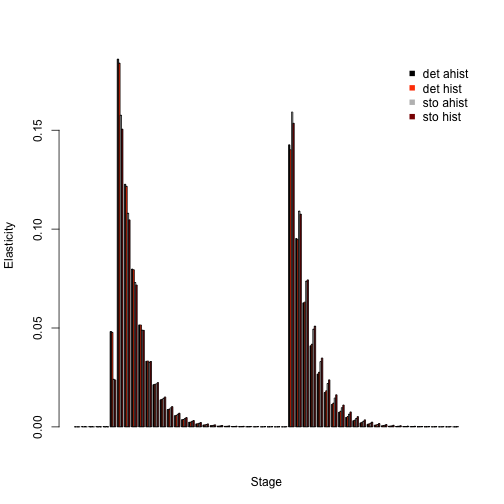Figure 5.8. Ahistorical vs. historically-corrected deterministic and stochastic elasticity to stage

Elasticity analyses in these plots look very similar. Population growth rates are most elastic in response to changes in stage 7, which is the 1-sprouted non-flowering adult stage. Small adult stages have higher elasticity than larger adults and juveniles. Most of the differences between analyses here appear to lie in elasticity of small non-flowering vs. flowering adults in the ahistorical vs. historical comparison.

Finally, let’s take a look at how the importance of different kinds of transitions changes, by looking at elasticity sums. The plot will show that population growth rates are most elastic in response to changes in growth and shrinkage, with virtually no influence of fecundity.

tm2elas_sums <- summary(tm2elas)
tm3elas_sums <- summary(tm3elas)
tm2elas_s_sums <- summary(tm2elas_s)
tm3elas_s_sums <- summary(tm3elas_s)

elas_sums_together <- cbind.data.frame(tm2elas_sums$ahist[,2], tm3elas_sums$ahist[,2], tm2elas_s_sums$ahist[,2], tm3elas_s_sums$ahist[,2])
names(elas_sums_together) <- c("det ahist", "det hist", "sto ahist", "sto hist")
rownames(elas_sums_together) <- tm2elas_sums$ahist$category

barplot(t(elas_sums_together), beside=T, ylab = "Elasticity", xlab = "Transition",
col = c("black", "orangered", "grey", "darkred"), bty = "n")
legend("topright", c("det ahist", "det hist", "sto ahist", "sto hist"),
col = c("black", "orangered", "grey", "darkred"), pch = 15, bty = "n")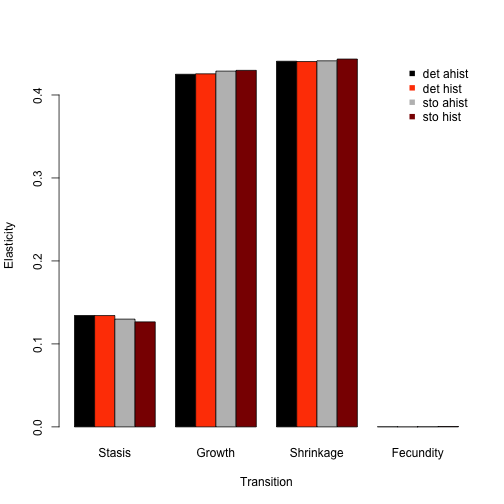Figure 5.9. Ahistorical vs. historically-corrected elasticity of lambda to transitions

Some users of lefko3 may wish to use conduct custom projections, perhaps involving density dependence or projected individual covariate inputs. Beginning with version 5.0, lefko3 includes function f_projection3(), which allows users to conduct projection simulations of function-based MPMs. This function takes the vital rate models that constitute the lefkoMod object input into matrix generating functions flefko3(), flefko2(), aflefko2(), and afleslie(), and builds matrices projecting the population forward according to whatever inputs the user has in mind. Function f_projection3() is different from function projection3() in that the latter requires a lefkoMat object as input, and uses the matrices in whatever projection the user has in mind. The former actually creates these matrices from scratch at each time step and so does not take matrices as inputs.

To illustrate how we might use this function, let’s run a conservation-focused analysis using the ahistorical vital rate models created above. We will ask what happens if we artificially pollinate these plants enough to double their fecundity. Let’s first create a lefkoDens object, which holds the density dependence information. Let’s also create an alternative supplement table with doubled fecundity.

c2d <- density_input(cypmatrix2, stage3 = c("SD", "P1", "SD", "P1"),
stage2 = c("SD", "SD", "rep", "rep"), style = 1,
time_delay = 1, alpha = 1, beta = 0, type = c(1, 1, 2, 2))
#c2d

cypsupp2alt <- supplemental(stage3 = c("SD", "P1", "P2", "P3", "SL", "SL", "D",
"V1", "V2", "V3", "SD", "P1"),
stage2 = c("SD", "SD", "P1", "P2", "P3", "SL", "SL", "SL", "SL", "SL", "rep",
"rep"),
eststage3 = c(NA, NA, NA, NA, NA, NA, "D", "V1", "V2", "V3", NA, NA),
eststage2 = c(NA, NA, NA, NA, NA, NA, "D", "D", "D", "D", NA, NA),
givenrate = c(0.08, 0.1, 0.1, 0.1, 0.125, 0.2, NA, NA, NA, NA, NA, NA),
multiplier = c(NA, NA, NA, NA, NA, NA, NA, NA, NA, NA, 0.5*2, 0.5*2),
type = c("S", "S", "S", "S", "S", "S", "S", "S", "S", "S", "R", "R"),
stageframe = cypframe, historical = FALSE)
#cypsupp2alt

Now let’s run two projections, the first using the original ahistorical information, and the second using the altered supplement table. We will run both as stochastic and density dependent, with ten replicates of each and 100 time steps.

set.seed(42)
basetrial <- f_projection3(format = 3, data = vertdata, supplement = cypsupp2,
stageframe = cypframe, modelsuite = cypmodels2, nreps = 10, times = 100,
stochastic = TRUE, growthonly = TRUE, substoch = 2, density = c2d)
#> Warning: Option patch not set, so will set to first patch/population.
alttrial <- f_projection3(format = 3, data = vertdata, supplement = cypsupp2alt,
stageframe = cypframe, modelsuite = cypmodels2, nreps = 10, times = 100,
stochastic = TRUE, growthonly = TRUE, substoch = 2, density = c2d)
#> Warning: Option patch not set, so will set to first patch/population.

#summary(basetrial)
#summary(alttrial)

Let’s compare these two projections with plots.

par(mfrow = c(1,2))
plot(basetrial, ylim = c(0, 100))
plot(alttrial, ylim = c(0, 100))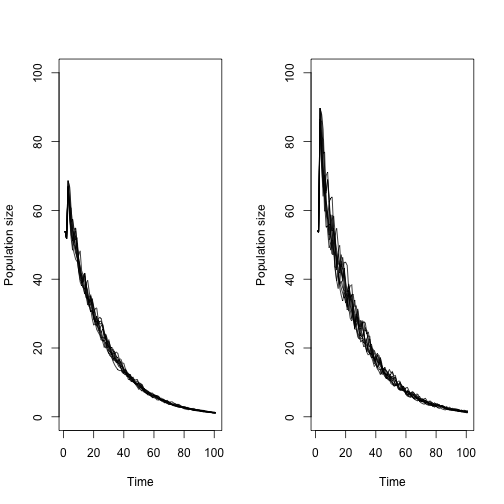Figure 5.10. Projected Cypripedium population dynamics against boosted fecundity

Each plot shows 10 lines, one for each replicate. Both projections show decline, but the population appears to reach a higher high size early on under the boosted fecundity scenario. So, while boosting fecundity does not prevent extinction, it does seem to boost the population and probably lowers the overall chances of extinction within a short time window.

We have also created other literature to help users familiarize themselves with population analyses in general and using the package. Users should see our free e-manual called lefko3: a gentle introduction, as well as other vignettes including long-format and video vignettes, on the projects page of the Shefferson lab website.

## Acknowledgements

We are grateful to Joyce and George Proper, Ken Klick, and the many other volunteers who have helped amass the Cypripedium dataset, as well as to two anonymous reviewers whose scrutiny improved the quality of this vignette. The project resulting in this package and this tutorial was funded by Grant-In-Aid 19H03298 from the Japan Society for the Promotion of Science.

## Literature cited

Bartoń, Kamil A. 2014. MuMIn: Multi-Model Inference.” https://CRAN.R-project.org/package=MuMIn.
Burnham, Kenneth P., and David R. Anderson. 2002. Model Selection and Multimodel Inference: A Practical Information-Theoretic Approach. New York, New York, USA: Springer-Verlag New York, Inc.
Ehrlén, Johan. 2000. “The Dynamics of Plant Populations: Does the History of Individuals Matter?” Ecology 81 (6): 1675–84. https://doi.org/10.1890/0012-9658(2000)081[1675:TDOPPD]2.0.CO;2.
Ellner, Stephen P., and Mark Rees. 2006. “Integral Projection Models for Species with Complex Demography.” American Naturalist 167 (3): 410–28. https://doi.org/10.1086/499438.
Shefferson, Richard P., Ryo Mizuta, and Michael J. Hutchings. 2017. “Predicting Evolution in Response to Climate Change: The Example of Sprouting Probability in Three Dormancy-Prone Orchid Species.” Royal Society Open Science 4 (1): 160647. https://doi.org/10.1098/rsos.160647.
Shefferson, Richard P., Brett K. Sandercock, Joyce Proper, and Steven R. Beissinger. 2001. “Estimating Dormancy and Survival of a Rare Herbaceous Perennial Using Mark-Recapture Models.” Ecology 82 (1): 145–56. https://doi.org/10.1890/0012-9658(2001)082[0145:EDASOA]2.0.CO;2.
Shefferson, Richard P., Robert J. Warren II, and H. Ronald Pulliam. 2014. “Life History Costs Make Perfect Sprouting Maladaptive in Two Herbaceous Perennials.” Journal of Ecology 102 (5): 1318–28. https://doi.org/10.1111/1365-2745.12281.